# MCQs on Power Systems

##### Page 12 of 67. Go to page 1 2 3 4 5 6 7 8 9 10 11 12 13 14 15 16 17 18 19 20 21 22 23 24 25 26 27 28 29 30 31 32 33 34 35 36 37 38 39 40 41 42 43 44 45 46 47 48 49 50 51 52 53 54 55 56 57 58 59 60 61 62 63 64 65 66 67
01․ A 1-phase transmission line has an impedance (4 + 3j ) Ω. Find the power factor of the load for zero voltage regulation?
0.6 lag
0.8 lag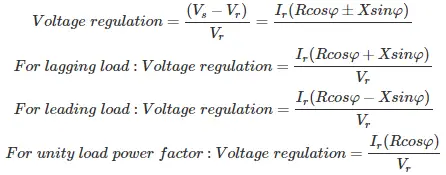Therefore, zero voltage regulation is possible only for leading power factor.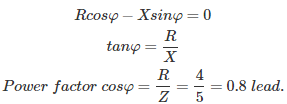02․ A 1-phase transmission line has an impedance (4 + 3j ) Ω. Find the power factor of the load for zero voltage regulation?
0.6 lagTherefore, zero voltage regulation is possible only for leading power factor.03․ Which of the following transmission line is most practically used in load flow analysis?
Nominal T
Nominal ∏
either 1 or 2
nether 1 nor 2

In nominal T network, the capacitance of the line is concentrated at the middle of the transmission line. Therefore it is difficult to find the external shunt capacitance to the line capacitance and also more equations are required. Therefore, it is not practically used in load flow analysis. In nominal ∏ network, line capacitance is equally distributed both at sending end and receiving end. By considering the line capacitance effect proper value of shunt capacitance can be designed. External shun capacitance can be easily combined with the line capacitance and also less number of equations are required. Therefore, it is most practically used as equivalent circuit of medium and long transmission line in load flow analysis.

04․ Transmission line has a length of 300 km, operating at sending end voltage Vs = 1 pu, find the receiving end voltage(Vr) at no load?
1 pu
0.98 pu
1.098 pu
0.098 pu

At no load, due to Ferranti effect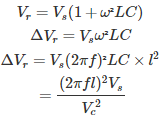Where, l = length of transmission line Vc = velocity of signal = 3×108 L = inductance of line C = capacitance of line f = frequency Therefore, ΔVr = 0.098 pu Receiving end voltage Vr = Vs + ΔVr = 1 + 0.098 = 1.098 pu

05․ A 3-phase transmission line operating at Vs = 400 kV and has ABCD parameters A= D = 0.8∠0°, B = 100∠°90 Ω, C = 0.5*10^-6∠90° â„§. Find Charging current under no load?
0.144∠90° A/phase
0.344∠90° A/phase
0.512∠90° A/phase
1.144∠90° A/phase

Using of ABCD parameters Vs = AVr + BIr Is = CVr + DIr Under no load condition Ir = 0, Is = charging current(Ic)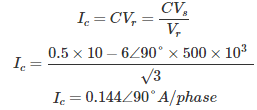06․ A 3-phase transmission line operating at Vs = 400 kV and has ABCD parameters A= D = 0.8∠0°, B = 100∠90° Ω, C = 0.5*10^-6∠90° â„§. Find the shunt reactor required for compensating ferranti effect?
1500 Ω
3000 Ω
500 Ω
2000 Ω

Inductive shunt reactance Xlsh = Vr/Ir Vs = AVr + BIr After compensation of ferranti effect Vs = Vr Vr = AVr + BIr BIr = (1-A)Vr Inductive shunt reactance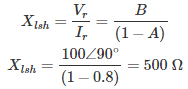07․ Installation of capacitors at suitable locations and of optimum size in a distribution system results in
improved voltage regulation
reduction in distribution power loss
reduction of KVA rating of distribution transformers
all of the above

Shunt capacitors are used with individual equipment to improve power factor. As KW = KVA×power factor The improved power factor reduces the KVA drawn from the supply. As power loss Pl = VI cosφ For fixed power loss and voltage, as power factor increases, I decreases. Therefore, I²R loss decrease. The power losses are reduced and the efficiency is increased. The smaller voltage drop in the line results in good voltage regulation. Thus, shunt capacitors regulate the voltage and reactive power flows at the points where they installed.

08․ Ferranti effect occurs under
any of the above

Under no load conditions or light load conditions of a transmission line, receiving end voltage is greater than sending ending voltage will occur in case of medium and transmission lines. This is called Ferranti effect. This is due to capacitance effect is dominating compare to inductance of transmission line under no load only. At no load, due to Ferranti effectWhere, l = length of transmission line Vc = velocity of signal = 3×108 L = inductance of line C = capacitance of line f = frequency

09․ A 3-phase transmission line operating at Vs = 400 kV and has ABCD parameters A= D = 0.8∠0°, B = 100∠90° Ω, C = 0.5*10-6∠90° â„§. Find the no load receiving end voltage?
400 kV
500 kV
600 kV
320 kv

Using of ABCD parameters Vs = AVr + BIr Is = CVr + DIr Under no load condition Ir = 0 Where, Vs = Sending end voltage Vr = Receiving end voltage Is = Sending end current Ir = Receiving end current Therefore,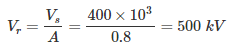10․ If transmission line parameters A and B are 0.9∠0° and 200∠90° respectively. Find the shunt reactor required to compensate the ferranti effect?
1500 Ω
3000 Ω
750 Ω
2000 Ω

Inductive shunt reactance Xsh = Vr/Ir Vs = AVr + BIr After compensation of ferranti effect Vs = Vr Vr = AVr + BIr BIr = (1-A)Vr Inductive shunt reactance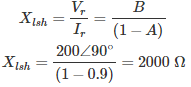<<<1011121314>>>